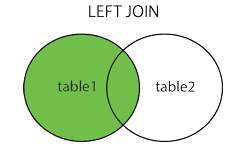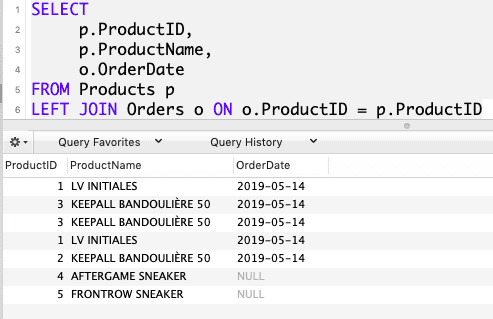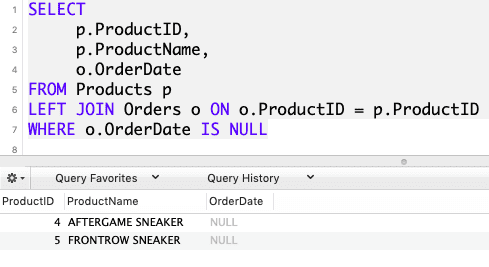# SQL LEFT OUTER JOIN: The Complete Guide

SQL LEFT JOIN clause allows us to query data from multiple tables. This means that the left join returns all the values from a left table, plus matched values from a right table or NULL in the case of no matching join predicate.

In some SQL databases, LEFT OUTER JOIN is also called LEFT JOIN.

## LEFT OUTER JOIN in SQL

SQL Left Outer Join returns all records from the left table (table1) and the matched records from the right table (table2). It returns all the rows from a left table and the matching rows from the right table.

### Syntax

The syntax of SQL Left Join is the following.

```SELECT column_name(s)
FROM table1
LEFT JOIN table2
ON table1.column_name = table2.column_name;```

In this syntax, table1 and table2 are the left and right tables, respectively.

For each row from the table1 table, the query compares it with all the rows from the table2 table.

If the pair of rows causes the join predicate to evaluate to true, the column values from these rows will be in the combined form to the new row, which is then included in the final result set.

If a row from the left table (table1) does not have any matching row from the table2 table, the query combines column values of a row from the left table with NULL for each column value from the right table.

In short, the LEFT JOIN clause returns all rows from a left table (table1) and matches the rows or NULL values from the right table (table2).

Venn Diagram of SQL Left Outer join is the following.### Example

We need to create two tables. Now, create the first table.

### SQL Create Tables

To create a table in SQL, use the CREATE TABLE statement. Run the following query to create the Products table.

```CREATE TABLE Products (
ProductID int PRIMARY KEY AUTO_INCREMENT,
ProductName varchar(255) NOT NULL,
ProductPrice int NOT NULL
);```

You can see the table in the database.

Now, create a second table using the following query.

```CREATE TABLE Orders (
OrderID int PRIMARY KEY AUTO_INCREMENT,
ProductID int NOT NULL,
OrderDate date NOT NULL
);```

So, we have created the Products and Orders table. Now, we need to fill the data into the tables.

### INSERT Data into Tables

The next thing to do is that we need to insert the data into the database.

Insert the data into the Products and Orders table using the INSERT INTO statement.

```INSERT INTO Products (`ProductName`,`ProductPrice`)
VALUES
('LV INITIALES', 60 ),
('KEEPALL BANDOULIÈRE 50', 70 ),
('KEEPALL BANDOULIÈRE 50', 80 ),
('AFTERGAME SNEAKER', 90 ),
('FRONTROW SNEAKER', 100 )```

Run the above code, and you will see the data is filled inside the Products with these values.

Now, we need to add Orders data. See, let’s do that. See the below query.

```INSERT INTO Orders (`ProductID`,`OrderDate`)
VALUES
(1, NOW() ),
(3, NOW() ),
(3, NOW() ),
(1, NOW() ),
(2, NOW() )```

We have used the SQL NOW() function to create current data.

Run the above query, and data is created inside the Orders table.

So we have created two tables with the data. Now, we will use SQL Left JOIN to query the data and fetch the results.

```SELECT
p.ProductID,
p.ProductName,
o.OrderDate
FROM Products p
LEFT JOIN Orders o ON o.ProductID = p.ProductID
```

So, in the above query, we are fetching three columns.

1. p.ProductID means in the Products table fetch the ProductID.
2. p.productName means in the Products table fetch the ProductName.
3. o,orderDate means in the Orders table fetch the OrderDate.

We have left Join Products with Orders table in which ProductID is common in both the tables.

In the Orders table, ProductID is a foreign key. Each sales order item includes one product. The link between the Products and the Orders tables is via the values in the ProductID column.

So, based on the foreign key, it maps the result in the final table.

In the final table, all the rows from the left table are included. In our case, the left table is Products.

So, all the Products table rows will be added, and on the right side, if the condition is specified, the rows will be included from the right table; otherwise, null will be returned for a particular column.

If you run the above query, you will find the following result.As you see clearly from the result set, a list of NULL in the OrderDate column indicates that the corresponding products have not been sold to any customer yet.

Now, you can also use the WHERE clause in the SQL query. Let’s fetch the record of ProductID and ProductName, whose OrderDate is NULL. See the below query.

```SELECT
p.ProductID,
p.ProductName,
o.OrderDate
FROM Products p
LEFT JOIN Orders o ON o.ProductID = p.ProductID
WHERE o.OrderDate IS NULL```

See the below result output image.So, we have seen how to create a table and then use the Left JOIN to fetch the records from two tables.

A left outer join can be used to return a result set that contains all rows in the first table that do not exist in the second table by testing in the WHERE clause the value of a NOT NULL column having a NULL value.

That’s it for this tutorial.

SQL Outer Join

SQL Right Join

SQL Self Join

SQL Cross Join

SQL Join

Categories SQL

This site uses Akismet to reduce spam. Learn how your comment data is processed.